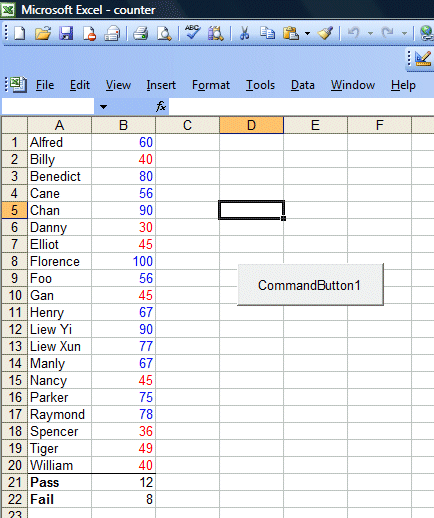Lesson 9: Creating a Counter in Excel VBA

This is a simple Excel VBA counter that can count the number of passes and the number of failures for a list of marks obtained by the students in an examination. The program can also differentiate the passes and failures with blue and red colors respectively. Let's examine the code below:

 Private Sub CommandButton1_Click() Dim i, counter As Integer For i = 1 To 20 If Cells(i, 2).Value > 50 Then counter = counter + 1 Cells(i, 2).Font.ColorIndex = 5 Else 'do nothing Cells(i, 2).Font.ColorIndex = 3 End If Next i Cells(21, 2).Value = counter Cells(22, 2).Value = 20 - counter End Sub

This program combines  For..Next and If ...Then...Else statements to control the program flow. If the value in that cell is more than 50, the value of counter is increased by 1  and the font color is changed to blue (the colorIndex is 5) , otherwise there is no increment in the counter and the font color is changed to red (ColorIndex=3). The output is as shown in Figure 9.1Figure 9.1